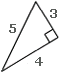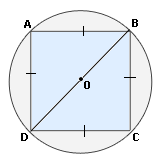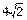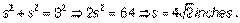## Definition Of Pythagorean Theorem

In a right triangle, if a and b are the measures of the legs and c is the measure of the hypotenuse, then a2 + b2 = c2.

### Example of Pythagorean Theorem

The right triangle shown has legs 3 and 4 and hypotenuse 5.### Solved Example on Pythagorean Theorem

#### Ques: The radius of the circle is 4 inches. Find the length of side of the square using Pythagorean theorem.##### Choices:

A. 4 inches
B. 8 inches
C.inches
D.inches
Step 5: Using Pythagorean Theorem,we get,